# Form Operators and Functions¶

Expressions in calculations, constraints, and relevants can contain operators and functions.

## Operators¶

### Math operators¶

Explanation Example
+ addition ${salary_income} +${self_employed_income}
- subtraction ${income} -${expenses}
* multiplication ${bill} * 1.18 div division${percent_int} div 100
mod modulo (division remainder) (${even_number} mod 2) = 0 Warning Math operators only work with numbers. ### Comparison operators¶ Comparison operators are used to compare values. The result of a comparison is always True or False. Explanation Example Notes = equal to${enrolled} = 'yes' Can compare numbers or strings.
!= not equal to ${enrolled} != 'yes' Can compare numbers or strings. > greater than${age} > 17
>= greater than or equal to ${age} >= 18 < less than${age} < 65
<= less than or equal to ${age} <= 64 Warning ### Boolean operators¶ Boolean operators combine two True or False values into a single True or False value. Explanation Example and True if the expressions before and after are True${age} > -1 and ${age} < 120 or True if either of the expressions before or after are True${age} < 19 or ${age} > 64 ### Path operators¶ Explanation Example Notes . current question's value . >= 18 Used in constraints. .. current question's parent group position(..) Used with position() to get a parent repeat instance's index. Note Formally, these are not operators but rather XPath references to the current node (.) and the parent node (..). XPath paths can be used to reference nodes of a form. ## Functions¶ ### Control flow¶ if(expression, then, else) Returns then if expression evaluates to True. Otherwise, returns else. position(xpath) Returns an integer equal to the 1-indexed position of the current node within the node defined by xpath. Most often this is used in the form position(..) to identify the current iteration index within a repeat group. XLSForm type name label repeat_count calculation note person_list_note Please list the names of the people in your household. begin_repeat person Member of household text name Name end_repeat begin_repeat person_details Details count(${person})
calculate current_name     indexed-repeat(${name},${person}, position(..))
date member_bday Birthday of ${current_name} end_repeat once(expression) Returns the value expression if the question's value is empty. Otherwise, returns the current value of the question. This can be used to ensure that a random number is only generated once, or to store the first value entered for a question in a way that is retrievable even if the response is changed later. Warning This function is often misunderstood. Read when expressions are evaluated to learn more. ### Accessing response values¶ Note The response from most question types can be accessed using variables. Functions are needed for accessing responses to multi select questions and questions inside repeat groups. #### Select questions¶ selected(space_delimited_array, string) Returns True if string is a member of space_delimited_array, otherwise returns False. Commonly used to determined if a specific choice was selected in a select question. (This is possible because a reference to a select question returns a space-delimited array of choice names.) XLSForm survey type name label hint relevant constraint select_multiple medical_issues what_issues Have you experienced any of the following? Select all that apply. select_multiple cancer_types what_cancer What type of cancer have you experienced? Select all that apply. selected(${what_issues}, 'cancer')
select_multiple diabetes_types what_diabetes What type of diabetes do you have? Select all that apply. selected(${what_issues}, 'diabetes') begin_group blood_pressure Blood pressure reading selected(${what_issues}, 'hypertension')
integer systolic_bp Systolic     . > 40 and . < 400
integer diastolic_bp Diastolic     . >= 20 and . <= 200
end_group
text other_health List other issues.   selected(${what_issues}, 'other') note after_health_note This note is after all health questions. choices list_name name label medical_issues cancer Cancer medical_issues diabetes Diabetes medical_issues hypertension Hypertension medical_issues other Other cancer_types lung Lung cancer cancer_types skin Skin cancer cancer_types prostate Prostate cancer cancer_types breast Breast cancer cancer_types other Other diabetes_types type_1 Type 1 (Insulin dependent) diabetes_types type_2 Type 2 (Insulin resistant) selected-at(space_delimited_array, n) Returns the string at the nth position of the space_delimited_array. (The array is zero-indexed.) Returns an empty string if the index does not exist. This can be used to get the name of a selected choice from a multi-select question. (This is possible because a reference to a select question returns a space-delimited array of choice names.) Note If used to get a choice name from a select question, this function returns the name, not the label, of the selected choice. To get the label in the current language, use jr:choice-name().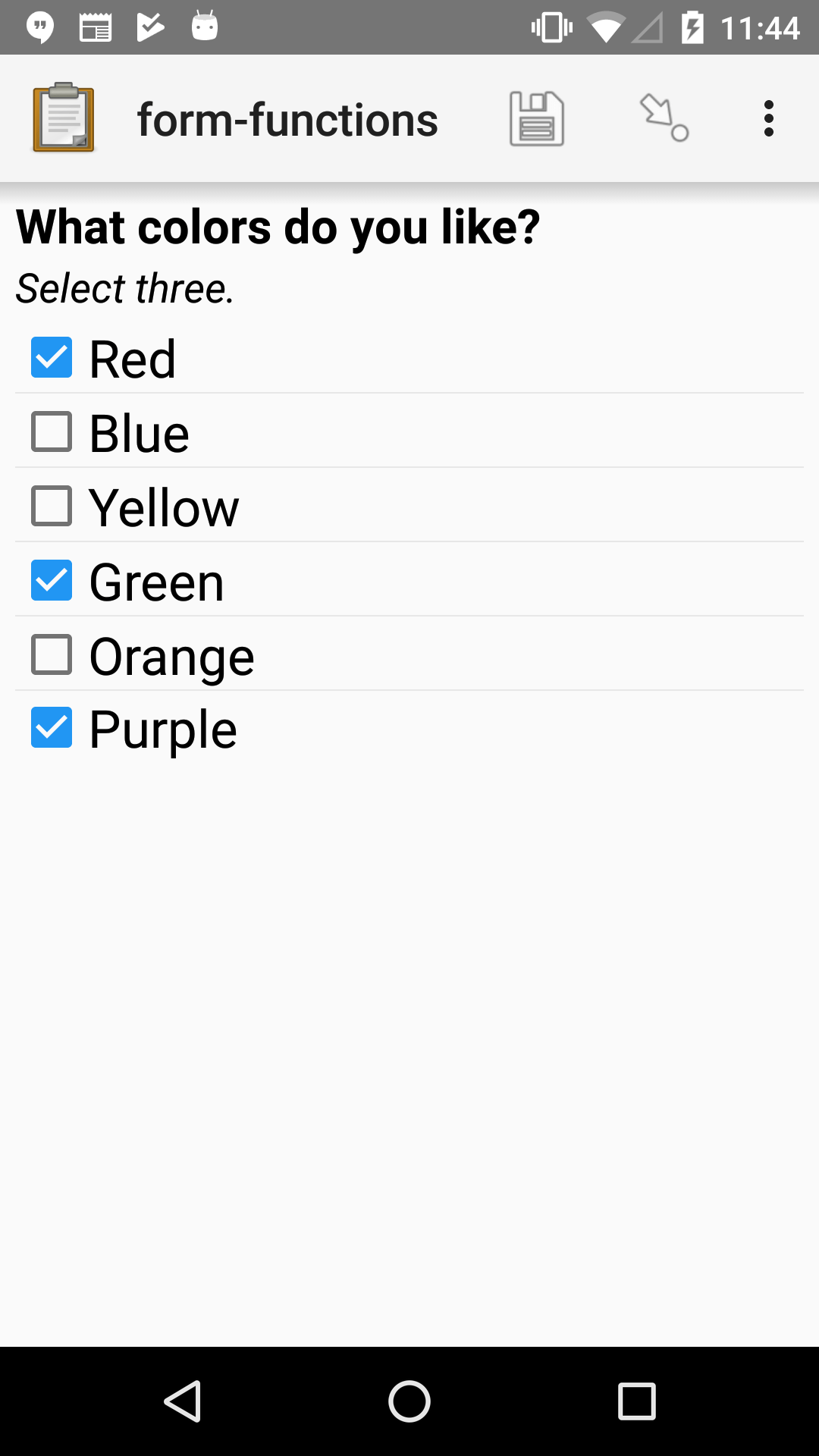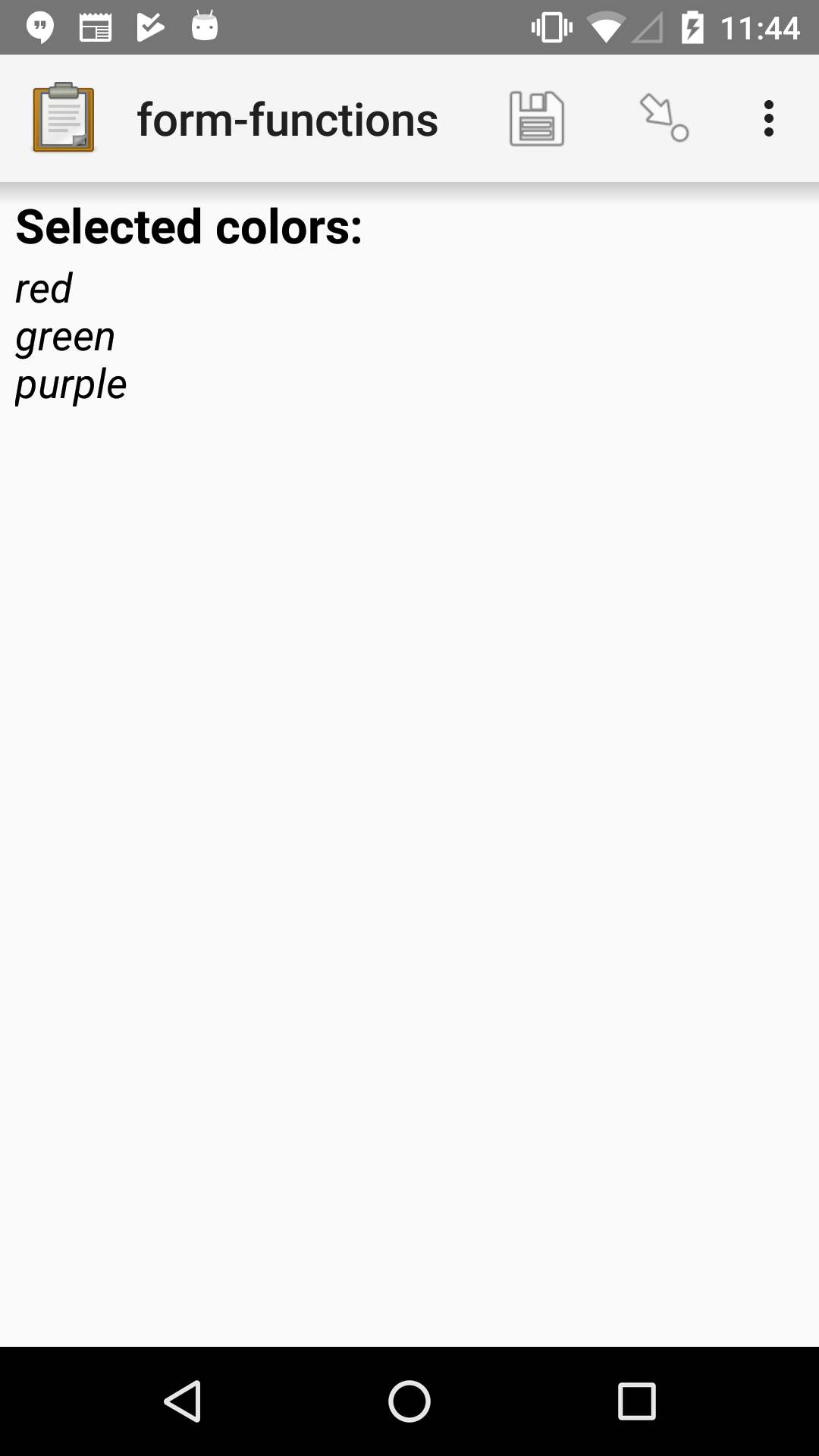XLSForm survey type name label hint calculation select_multiple colors color_prefs What colors do you like? Select three. calculate color_0 selected-at(${color_prefs}, 0)
calculate color_1     selected-at(${color_prefs}, 1) calculate color_2 selected-at(${color_prefs}, 2)
note color_note Selected colors: ${color_0} <br>${color_1} <br> ${color_2} choices list_name name label colors red Red colors blue Blue colors yellow Yellow colors green Green colors orange Orange colors purple Purple count-selected(multi_select_question) Returns the number of choices selected in multi_select_question.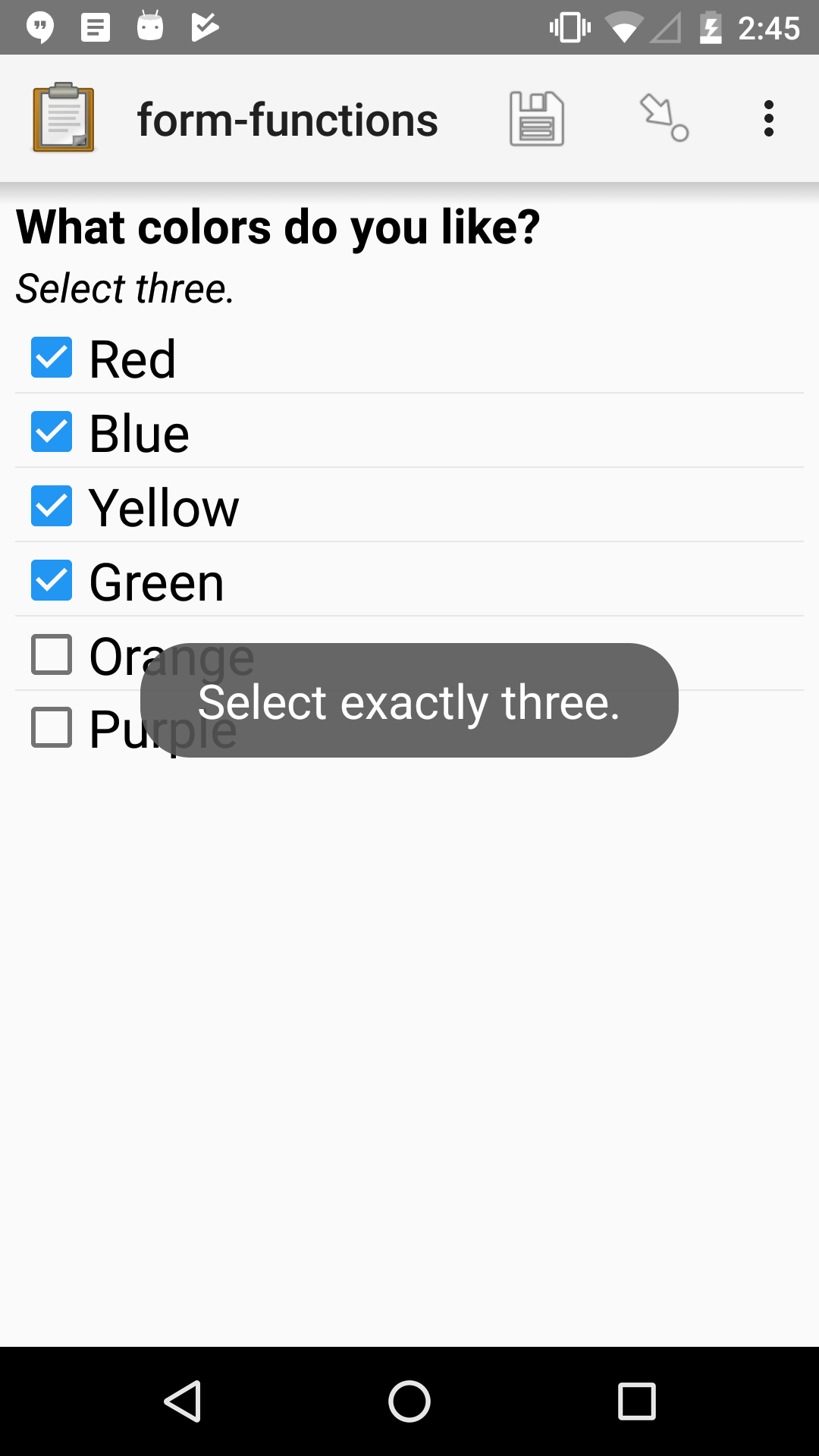XLSForm survey type name label hint constraint constraint_message select_multiple colors color_prefs What colors do you like? Select three. count-selected(.)=3 Select exactly three. choices list_name name label colors red Red colors blue Blue colors yellow Yellow colors green Green colors orange Orange colors purple Purple jr:choice-name(choice_name, 'select_question') Returns the label value, in the active language, associated with the choice_name in the list of choices for the select_question. Note You have to wrap the select_question reference in quotes. '${question_name}'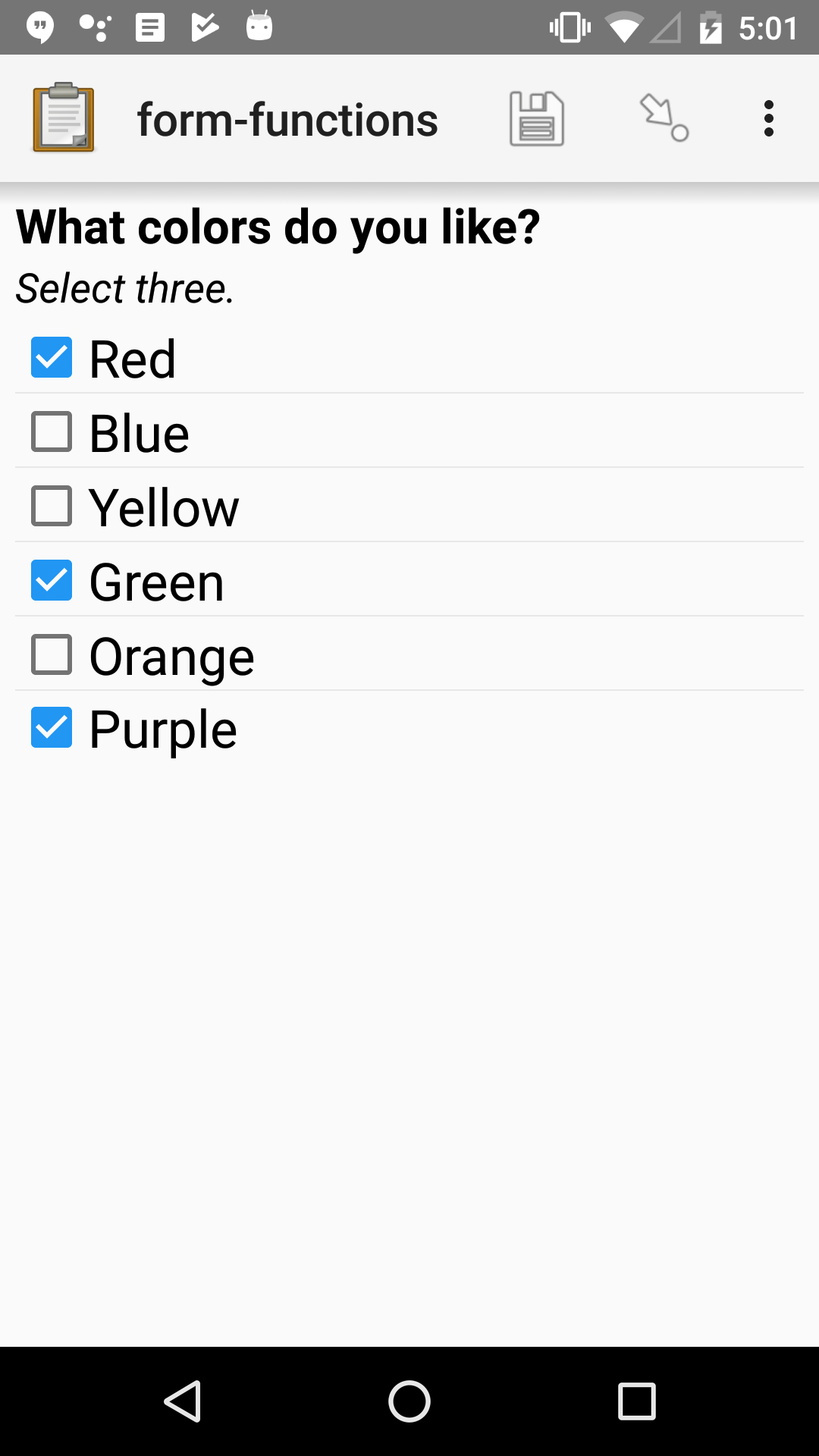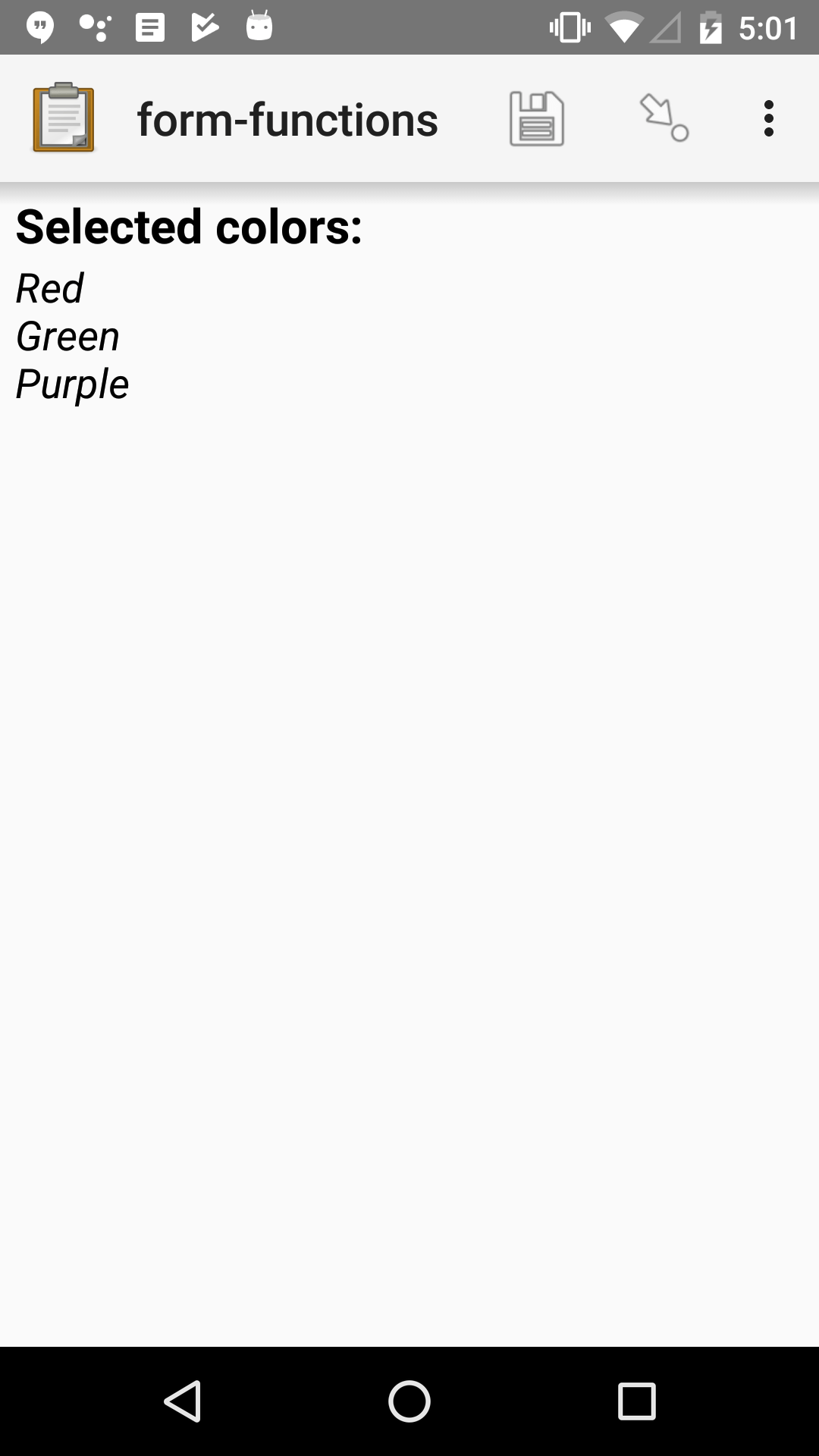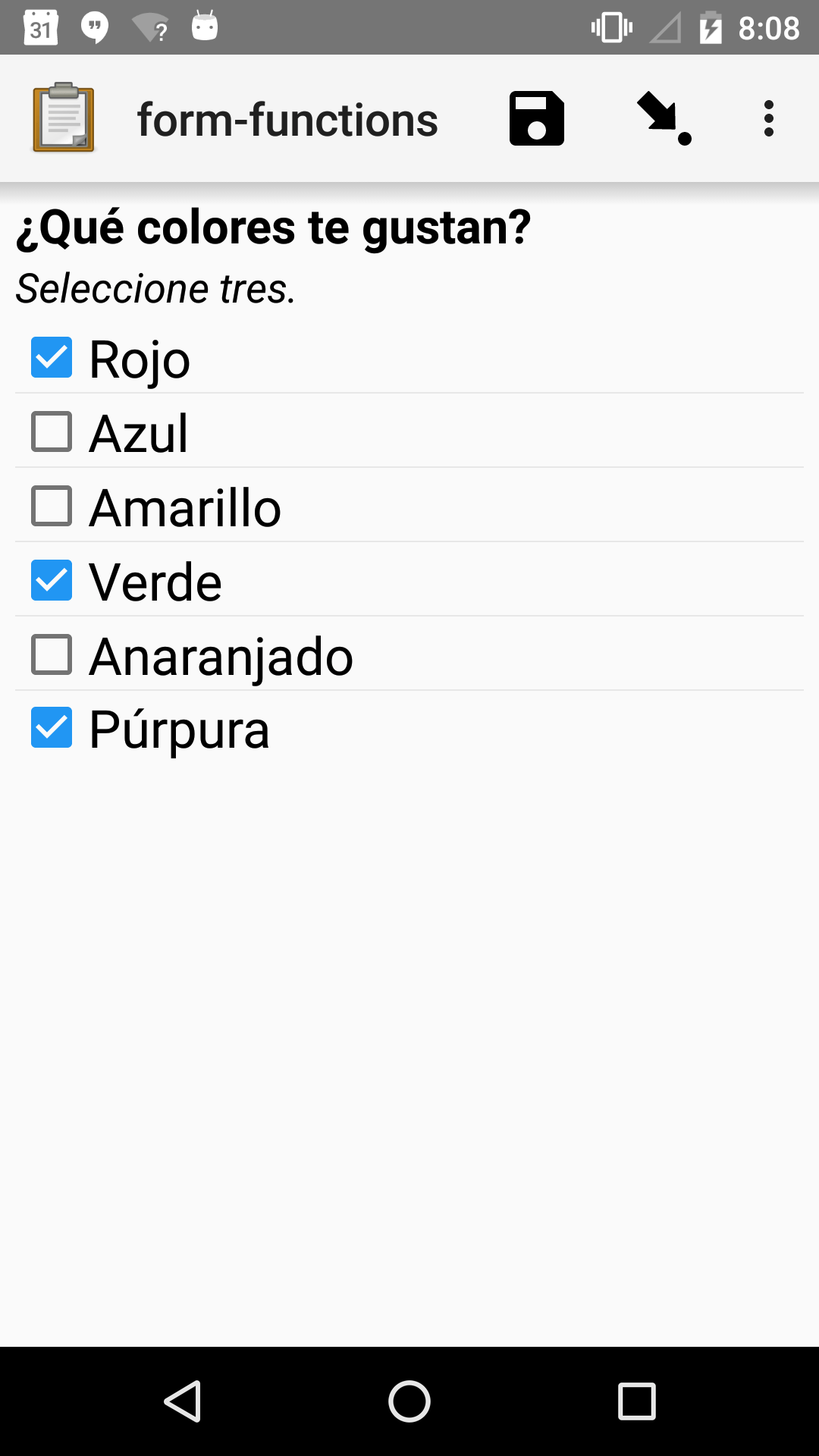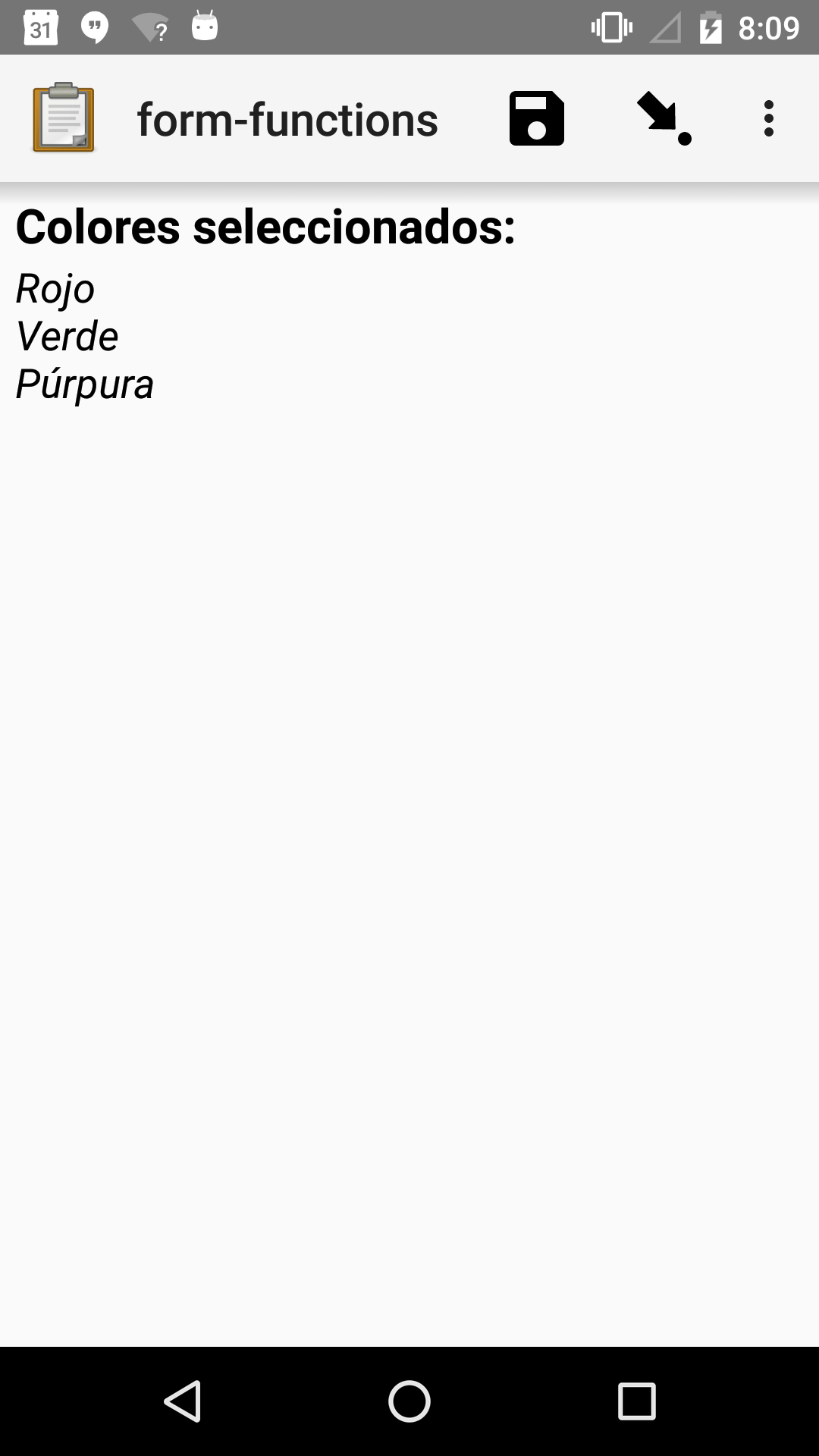XLSForm

survey
type name label::English label::Español hint::English hint:Español calculation
select_multiple colors color_prefs What colors do you like? ¿Qué colores te gustan? Select three. Seleccione tres.
calculate color_0         jr:choice-name( selected-at(${color_prefs}, 0), '${color_prefs}')
calculate color_1         jr:choice-name( selected-at(${color_prefs}, 1), '${color_prefs}')
calculate color_2         jr:choice-name( selected-at(${color_prefs}, 2), '${color_prefs}')
note color_note Selected colors: Colores seleccionados: ${color_0} <br>${color_1} <br> ${color_2}${color_0} <br> ${color_1} <br>${color_2}
choices
list_name name label::English label::Español
colors red Red Rojo
colors blue Blue Azul
colors yellow Yellow Amarillo
colors green Green Verde
colors orange Orange Anaranjado
colors purple Purple Púrpura

#### Repeat groups¶

Helpful terms

nodeset

A collection of XML nodes. In XLSForms, this is typically a collection of response values.

Outside a repeat group, referring to a question by name will return a nodeset containing all the responses to that question.

Nodesets can also be created by joining two or more nodes with pipes: /data/age | /data/name.

indexed-repeat(name, group, i[, sub_grp, sub_i[, sub_sub_grp, sub_sub_i]])

Returns the response value of question name from the repeat-group group, in iteration i.

Nested repeat groups can be accessed using the sub and sub_sub parameters.

XLSForm

type name label repeat_count calculation
note person_list_note Please list the names of the people in your household.
begin_repeat person Member of household
text name Name
end_repeat
begin_repeat person_details Details count(${person}) calculate current_name indexed-repeat(${name}, ${person}, position(..)) date member_bday Birthday of${current_name}
end_repeat
count(nodeset)

Returns the number of items in nodeset. This can be used to count the number of repetitions in a repeat group.

XLSForm

type name label repeat_count calculation
note person_list_note Please list the names of the people in your household.
begin_repeat person Member of household
text name Name
end_repeat
begin_repeat person_details Details count(${person}) calculate current_name indexed-repeat(${name}, ${person}, position(..)) date member_bday Birthday of${current_name}
end_repeat
count-non-empty(nodeset)

Returns the number of non-empty members of nodeset.

sum(nodeset)

Returns the sum of the members of nodeset.

Can be used to tally responses to a repeated select question.

XLSForm

survey
type name label calculation
begin_repeat guest_details Guest details
text guest_name Guest name
select_one meal_options meal_preference Meal preference
calculate chkn   if(${meal_preference} = 'chicken', 1, 0 ) calculate fsh if(${meal_preference} = 'fish', 1, 0 )
calculate veg   if(${meal_preference} = 'vegetarian', 1, 0 ) end_repeat calculate chkn_count sum(${chkn})
calculate fsh_count   sum(${fsh}) calculate veg_count sum(${veg})
choices
list_name name label
meal_options chicken Chicken
meal_options fish Fish
meal_options vegetarian Vegetarian
max(nodeset)

Returns the largest member of nodeset.

XLSForm

survey
type name label calculation
begin_repeat child_questions Questions about child
text child_name Child's name
integer child_age Child's age
end_repeat
calculate age_of_oldest_child   max(${child_age}) min(nodeset) Returns the smallest member of nodeset. XLSForm survey type name label calculation begin_repeat child_questions Questions about child text child_name Child's name integer child_age Child's age end_repeat calculate age_of_youngest_child min(${child_age})

Warning

The min() and max() functions only work sets of numbers. Empty values (that is, variables referencing unanswered questions) are actually empty strings, and will not be automatically converted to zero (0).

### Strings¶

#### Searching and matching strings¶

regex(string, expression)
Returns True if string is an exact and complete match for expression.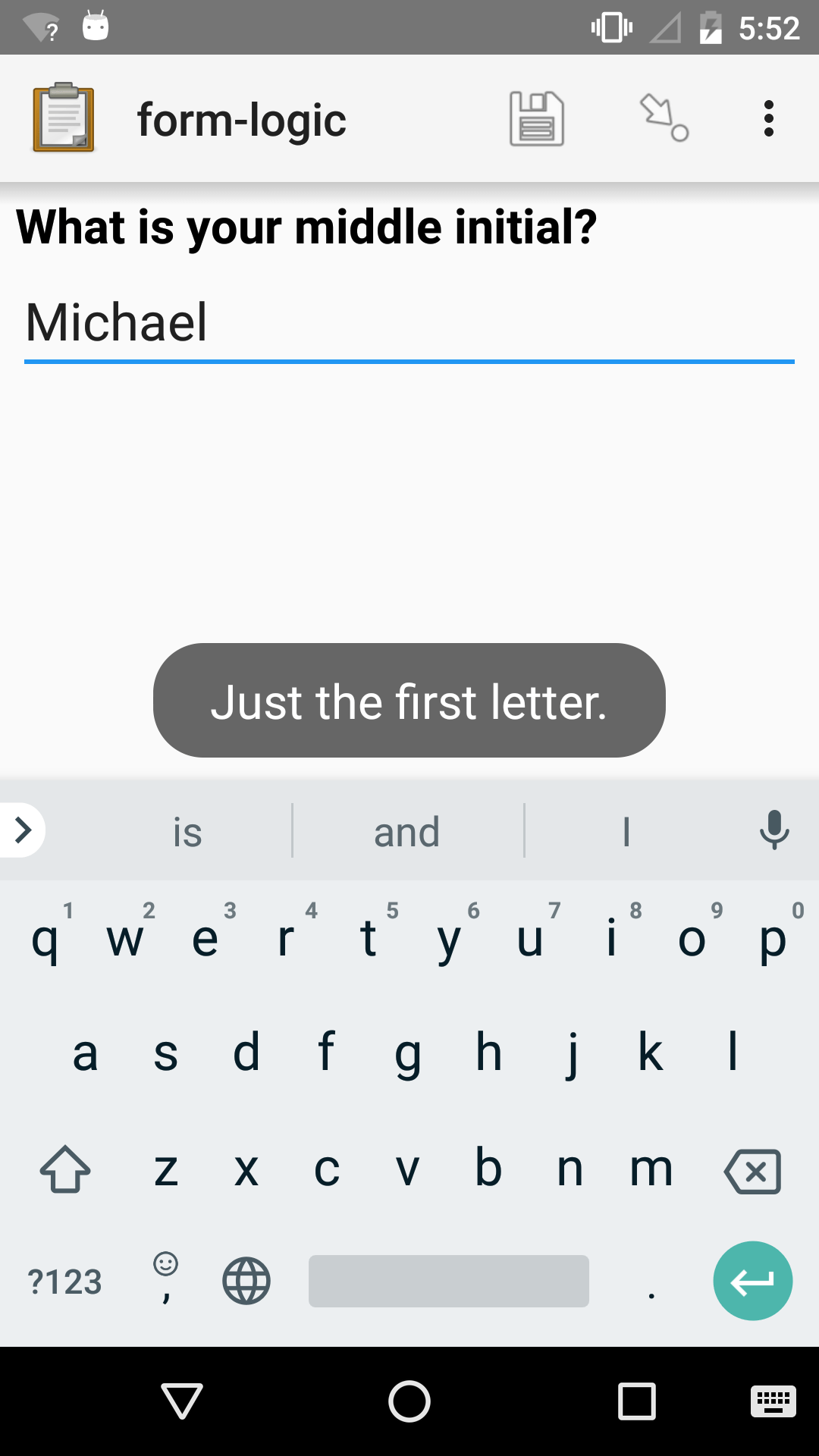XLSForm

survey
type name label constraint constraint_message
text middle_initial What is your middle initial? regex(., 'p{L}') Just the first letter.
contains(string, substring)

Returns True if the string contains the substring.

starts-with(string, substring)

Returns True if string begins with substring.

ends-with(string, substring)

Returns True if the string ends with substring.

substr(string, start[, end])

Returns the substring of string beginning at the index start and extending to (but not including) index end (or to the termination of string, if end is not provided). Members of string are zero-indexed.

substring-before(string, target)

Returns the substring of string before the first occurrence of the target substring. If the target is not found, or string begins with the target substring, then this will return an empty string.

substring-after(string, target)

Returns the substring of string after the first occurrence of the target substring. If the target is not found this will return an empty string.

translate(string, fromchars, tochars)

Returns a copy of string, where every occurrence of a character in fromchars is replaced by the corresponding character in tochars. If fromchars is longer than tochars then every occurrence of a character in fromchars that does not have a corresponding character in tochars will be removed.

string-length(string)

Returns the number of characters in string. If no value is passed in, returns the number of characters in the value of the question that this function call is tied to which can be useful in a constraint expression.

normalize-space(string)

Returns a string with normalized whitespace by stripping leading and trailing whitespace of string and replacing sequences of whitespace characters with a single space. If no value is passed in, normalizes whitespace of the value of the question that this function call is tied to.

#### Combining strings¶

concat(arg [, arg [, arg [, arg [...]]]])

Concatenates one or more arguments into a single string. If any arg is a nodeset, the values within the set are concatenated into a string.

join(separator, nodeset)

Joins the members of nodeset, using the string separator.

#### Converting to and from strings¶

boolean-from-string(string)

Returns True if string is "true" or "1". Otherwise, False.

string(arg)

Converts arg to a string.

### Math¶

Warning

Math functions (except number()) only work with number values.

You can use number() to convert a string of digits to a number, but it is usually better to get a number value directly.

Empty values (that is, variables referencing unanswered questions) are actually empty strings, and will not be automatically converted to zero (0).

#### Number handling¶

round(number, places)

Rounds a decimal number to some number of decimal places.

int(number)

Truncates the fractional portion of a decimal number to return an integer.

number(arg)

Converts arg to number value.

If arg is a string of digits, returns the number value.

If arg is True, returns 1. If arg is False, returns 0.

If arg cannot be converted, returns NaN (not a number).

digest(data, algorithm, encoding method (optional))

Computes and returns the hash value of the data string using the indicated hash algorithm string, and encoding this hash value using the optional encoding string.

Options for the algorithm are MD5, SHA-1, SHA-256, SHA-384, SHA-512.

If the third parameter is not specified, the encoding is base64. Valid options for the encoding are base64 and hex.

This function can be useful if, for example, someone wants to build a unique identifier from sensitive data like a national ID number without compromising that data.

#### Calculation¶

pow(number, power)

Raises a number to a power.

log(number)

Returns the natural log of number.

log10(number)

Returns the base-10 log of number.

abs(number)

Returns the absolute value of number.

sin(number)

Returns the sine of number.

cos(number)

Returns the cosine of number.

tan(number)

Returns the tangent of number.

asin(number)

Returns the arc sine of number.

acos(number)

Returns the arc cosine of number.

atan(number)

Returns the arctan of number.

atan2(y, x)

Returns the multi-valued inverse tangent of y, x.

sqrt(number)

Returns the square root of number.

exp(x)

Returns e^x.

exp10(x)

Returns 10^x.

pi()

Returns an approximation of the mathematical constant π.

### Date and time¶

today()

Returns the current date without a time component.

now()

Returns the current datetime in ISO 8601 format, including the timezone.

Warning

This function is often misused. Read when expressions are evaluated to learn more.

#### Converting dates and time¶

decimal-date-time(dateTime)

Converts dateTime value to the number of days since January 1, 1970 (the Unix Epoch).

This is the inverse of date().

date(days)

Converts an integer representing a number of days from January 1, 1970 (the Unix Epoch) to a standard date value.

This is the inverse of decimal-date-time().

decimal-time(time)

Converts time to a number representing a fractional day. For example, noon is 0.5 and 6:00 PM is 0.75.

#### Formatting dates and times for display¶

format-date(date, format)

Returns date as a string formatted as defined by format.

The following identifiers are used in the format string:

 %Y 4-digit year %y 2-digit year %m 0-padded month %n numeric month %b short text month (Jan, Feb, Mar…) %d 0-padded day of month %e day of month %a short text day (Sun, Mon, Tue…).

Note

Month and day abbreviations are language and locale specific. If form locale can be determined, that locale will be used. Otherwise, the device locale will be used.

format-date-time(dateTime, format)

Returns dateTime as a string formatted as defined by format.

The identifiers list in format-date() are available, plus the following:

 %H 0-padded hour (24-hr time) %h hour (24-hr time) %M 0-padded minute %S 0-padded second %3 0-padded millisecond ticks.

### Geography¶

area(nodeset | geoshape)

Returns the area, in square meters, of either a nodeset of geopoints or a geoshape value.

It takes into account the circumference of the Earth around the Equator but does not take altitude into account.

distance(nodeset | geoshape | geotrace)

Returns the distance, in meters, of either:

• a nodeset of geopoints
• the perimeter of a geoshape
• the length of a geotrace value

It takes into account the circumference of the Earth around the Equator and does not take altitude into account.

### Utility¶

random()

Returns a random number between 0.0 (inclusive) and 1.0 (exclusive).

Warning

This function is often misused. Read when expressions are evaluated to learn more.

randomize(nodeset[, seed])

Returns a shuffled nodeset.

A shuffle with a numeric seed is deterministic and reproducible.

The primary use for this function is to randomize the order of choices for a select question. The documentation on select widgets describes how this is done in XLSForm.

randomize() can only be used in a context where a nodeset is accepted. Note that questions of type calculate cannot reference a nodeset.

uuid([length])

Without argument, returns a random RFC 4122 version 4 compliant UUID.

With an argument it returns a random GUID of specified length.

boolean(arg)

Returns True if arg is:

• a number other than zero
• a non-empty string
• a non-empty collection
• a comparison or expressions that evaluates to True.

Returns False if arg is:

• the number 0
• an empty string
• an empty collection
• a comparison or expression that evaluates to False.
not(arg)

Returns the opposite of boolean(arg).

coalesce(arg, arg)

Returns first non-empty value of the two arg s. Returns an empty string if both are empty or non-existent.

checklist(min, max, response[, response[, response[, ...]]])

Returns True if the number of response s that are exactly the string "yes" is between min and max, inclusive.

Set min or max to -1 to make the argument not applicable.

weighted-checklist(min, max, reponse, weight[, response, weight[, response, weight[, response, weight[, ... ]]])

Returns True if the sum of the weight s of each response that is exactly the string "yes" is between min and max, inclusive.

Set min or max to -1 to make the argument not

true()

Evaluates to True.

false()

Evaluates to False.